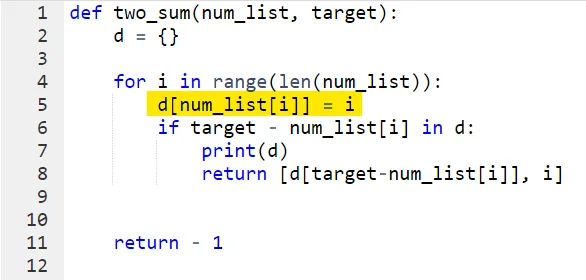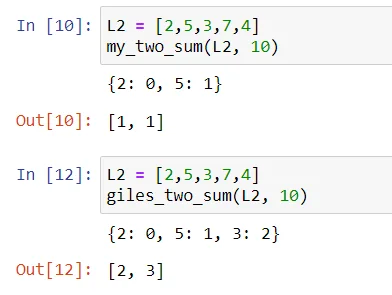29 Jun 2022

Posted on:

27 Jun 2022

0

# Resolved:Two_sum solution: Order of the lines of code

Hello,

The function returns a list containing the indices of the correct pair of numbers that would add up to the target value. So, does it mean that we print the dictionary d to serve as reference to check the pair of numbers from that result? I just noticed that the resulting dictionary doesn't include the last key-value pair of the 2nd index in the list.

Based on the above observation, does it affect the function in any other way if I put the d[nums[ i ]] = [ i ] above the if statement? Because when the code is executed in this way, the dictionary will always include the key-value pair of the 2nd index in the resulting list.
Also from Giles' sketch, he did created a dictionary first before comparing the pairs of numbers, so I think this is in line with his explanation.Thank you :)

Instructor
Posted on:

28 Jun 2022

0

Dear Carl,

Yes, printing out the dictionary serves only as a sanity check. In this way you make sure that the algorithm is performing as you expect it to. It is, therefore, not crucial for the solution to have both the first and the second addends printed out.

Your solution of putting `d[num_list[i]] = i` above the `if`-statement is absolutely correct but it switches the logic slightly. This can best be seen with the `two_sum(L2, 10)` example, where `L2` is defined as follows:

``````L2 = [2,5,3,7,4]
``````

Notice how Giles' solution and your solution return different (but correct) results. Can you figure out why this happens? :)

Kind regards,
365 Hristina

Posted on:

29 Jun 2022

0

Hello Hristina,

Now that you mentioned it, I've seen the difference. It seems that Giles' solution returns a unique pair of addends. However, my solution would return a different result if the target is an even number, such as this one:In my solution, the target is 10, so the 2nd iteration of the for loop will add the 5 to d (line 5). Then, by the time the code runs line 6, the if-statement `if target - num_list[i] in d `is now True, since 10 - 5 = 5 is indeed in d. And, since we are still in the 2nd iteration of the for loop, the return values would just be the same. Amazing!

A side note: I assumed that this different result will only occur when the target value is an even integer (target = 2 * num[i]), and/or the 7 and 3 came before 5 in L2 for this example.

Instructor
Posted on:

29 Jun 2022

0

Hey Carl,

Yes, absolutely - a discrepancy between the two approaches could occur only if the desired sum is even.

Keep up the good work!

Kind regards,
365 Hristina